# PSAT Math : How to find the slope of perpendicular lines

## Example Questions

← Previous 1

### Example Question #1 : How To Find The Slope Of Perpendicular Lines

Two points on line m are (3,7) and (-2, 5).  Line k is perpendicular to line m.  What is the slope of line k?

2/5

-5/2

0

-5

3

-5/2

Explanation:

The slope of line m is the (y2 - y1) / (x- x1) = (5-7) / (-2 - 3)

= -2 / -5

= 2/5

To find the slope of a line perpendicular to a given line, we must take the negative reciprocal of the slope of the given line.

Thus the slope of line k is the negative reciprocal of 2/5 (slope of line m), which is -5/2.

### Example Question #2 : How To Find The Slope Of Perpendicular Lines

The equation of a line is: 8x + 16y = 48

What is the slope of a line that runs perpendicular to that line?

2

-2

-1/8

8

-1/4

2

Explanation:

First, solve for the equation of the line in the form of y = mx + b so that you can determine the slope, m of the line:

8x + 16y = 48

16y = -8x + 48

y = -(8/16)x + 48/16

y = -(1/2)x + 3

Therefore the slope (or m) = -1/2

The slope of a perpendicular line is the negative inverse of the slope.

m = - (-2/1) = 2

### Example Question #3 : How To Find The Slope Of Perpendicular Lines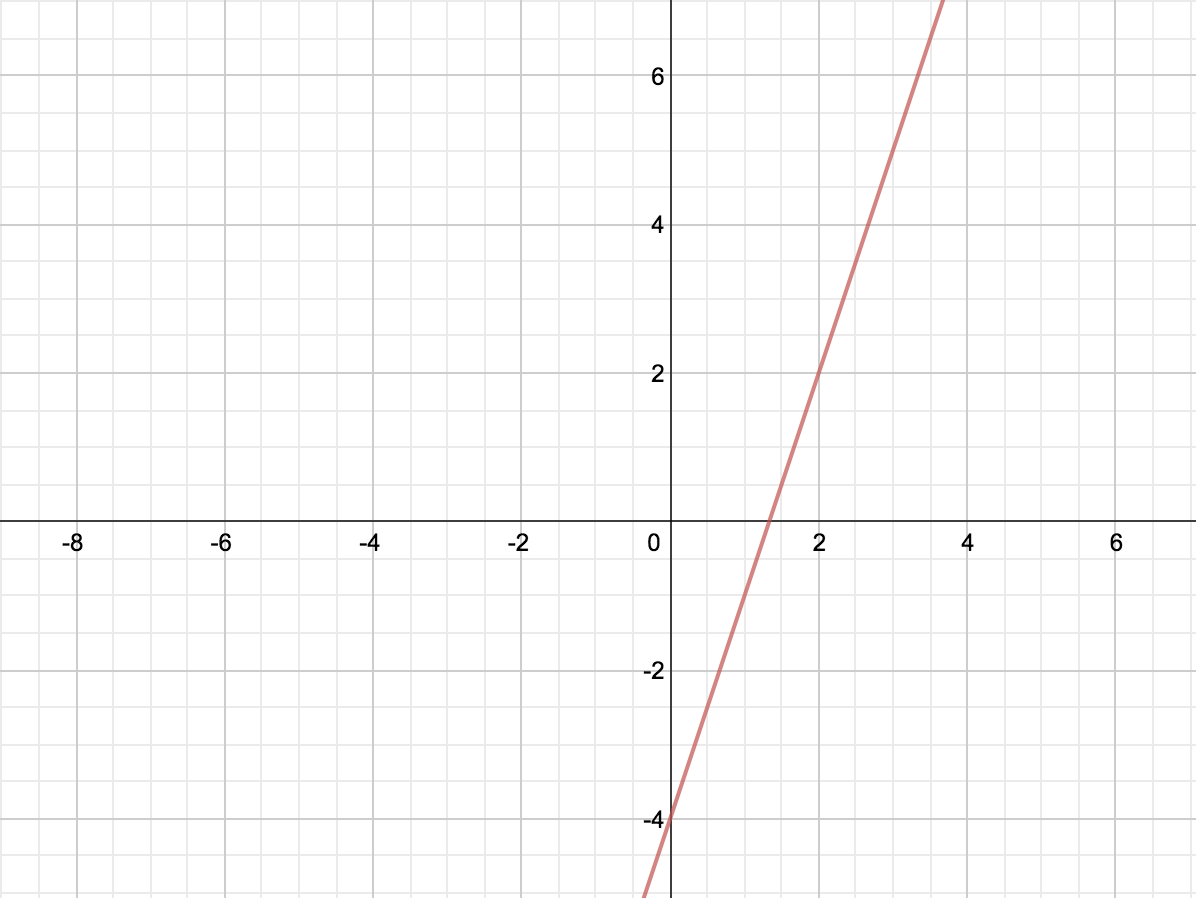What is the equation of a line perpendicular to the one above, passing through the point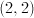?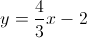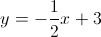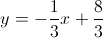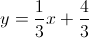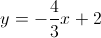Explanation:

Looking at the graph, we can tell the slope of the line is 3 with a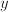-intercept of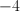, so the equation of the line is: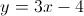A perpendicular line to this would have a slope of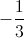, and would pass through the pointso it follows: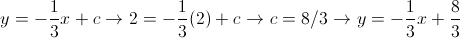### Example Question #4 : How To Find The Slope Of Perpendicular Lines

Line M passes through the points (2,2) and (3,–5).  Which of the following is perpendicular to line M?

y = 7x + 4

y = –7x – 5

y = (1/7)x + 3

y = –(1/7)x – 1

y = 7x – 6

y = (1/7)x + 3

Explanation:

First we find the slope of line M by using the slope formula (y– y1)/(x– x1).

(–5 – 2)/(3 – 2) = –7/1. This means the slope of Line M is –7.  A line perpendicular to Line M will have a negative reciprocal slope. Thus, the answer is = (1/7)x + 3.

### Example Question #1 : How To Find The Slope Of Perpendicular Lines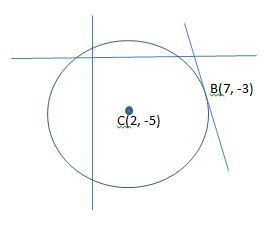Figure not drawn to scale.

In the figure above, a circle is centered at point C and a line is tangent to the circle at point B. What is the equation of the line?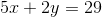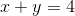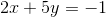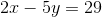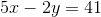Explanation:

We know that the line passes through point B, but we must calculate its slope in order to find the equation that defines the line. Because the line is tangent to the circle, it must make a right angle with the radius of the circle at point B. Therefore, the slope of the line is perpendicular to the slope of the radius that connects the center of the circle to point B. First, we can find the slope of the radius, and then we can determine the perpendicular slope.

The radius passes through points C and B. We can use the formula for the slope (represented as) between two points to find the slope of the radius.

Point C: (2,-5) and point B: (7,-3)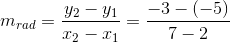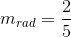This is the slope of the radius, but we need to find the slope of the line that is perpendicular to the radius. This value will be equal to the negative reciprocal.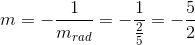Now we know the slope of the tangent line. We can use the point-slope formula to find the equation of the line. The formula is shown below.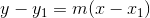Plug in the give point that lies on the tangent line (point B) and simplify the equation.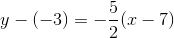Multiply both sides by two in order to remove the fraction.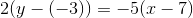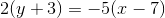Distribute both sides.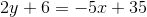Add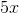to both sides.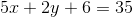Subtract six from both sides.The answer is.

### Example Question #1 : X And Y Intercept

Solve the equation for x and y.

x² + y = 31

x + y = 11

x = 13, 7

y = 8, –6

x = 6, 15

y = 5, –4

x = 8, –6

y = 13, 7

x = 5, –4

y = 6, 15

x = 5, –4

y = 6, 15

Explanation:

Solving the equation follows the same system as the first problem. However since x is squared in this problem we will have two possible solutions for each unknown. Again substitute y=11-x and solve from there. Hence, x2+11-x=31. So x2-x=20. 5 squared is 25, minus 5 is 20. Now we know 5 is one of our solutions. Then we must solve for the second solution which is -4. -4 squared is 16 and 16 –(-4) is 20. The last step is to solve for y for the two possible solutions of x. We get 15 and 6. The graph below illustrates to solutions.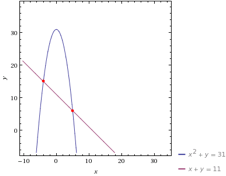### Example Question #2 : How To Find The Equation Of A Curve

Solve the equation for x and y.

x² – y = 96

x + y = 14

x = 15, 8

y = 5, –14

x = 25, 4

y = 10, –11

x = 5, –14

y = 15, 8

x = 10, –11

y = 25, 4

x = 10, –11

y = 25, 4

Explanation:

This problem is very similar to number 2. Derive y=14-x and solve from there. The graph below illustrates the solution.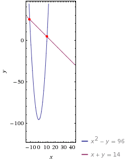### Example Question #3 : How To Find The Equation Of A Curve

Solve the equation for x and y.

5x² + y = 20

x² + 2y = 10

No solution

x = √10/3, –√10/3

y = 10/3

x = 14, 5

= 4, 6

x = √4/5, 7

= √3/10, 4

x = √10/3, –√10/3

y = 10/3

Explanation:

The problem involves the same method used for the rest of the practice set. However since the x is squared we will have multiple solutions. Solve this one in the same way as number 2. However be careful to notice that the y value is the same for both x values. The graph below illustrates the solution.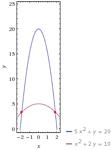### Example Question #4 : How To Find The Equation Of A Curve

Solve the equation for x and y.

x² + y = 60

x – y = 50

x = 40, 61

y = 11, –10

x = 11, –10

y = 40, 61

x = 10, –11

y = –40, –61

x = –40, –61

y = 10, –11

x = 10, –11

y = –40, –61

Explanation:

This is a system of equations problem with an x squared, to be solved just like the rest of the problem set. Two solutions are required due to the x2. The graph below illustrates those solutions.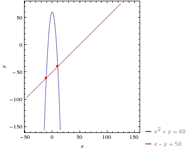### Example Question #5 : How To Find The Equation Of A Curve

A line passes through the points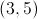and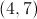. What is the equation for the line?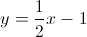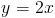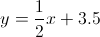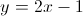Explanation:

First we will calculate the slope as follows: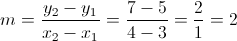And our equation for a line is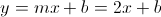Now we need to calculate b. We can pick either of the points given and solve for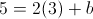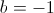Our equation for the line becomes← Previous 1